# NCERT Circles Class 10 Exercise 10.2 Solution

Theorem 10.2

prove that ” The Lengths of tangents drawn from an external points to a circle are equal.”

Given:

C ( O , r )

P = External point from the circle

P A and P B are tangents From External Point P

Construction:

Join External Point P , Point of contacts A and B to Center O .

Proof:

In Δ PAO and Δ PBO

P O = P O { Common Sides }

O A = O B { Radii Of Same Circle }

∠PAO = ∠PBO = 90º { Radius is always perpendicular (⊥) on Point of contact of tangent by Theorem 10.1 }

Δ PAO ≅ Δ PBO { By R.H.S. }

P A = P B { By CPCT }

Hence Proved

Key Points

• ∠OPA = ∠OPB { By CPCT }
• ∠POA = ∠POB  { By CPCT }
• P O is the angle bisector of ∠APB and ∠AOB

## Exercise – 10.2

In Question 1 to 3 , Choose the correct option and give justification.

Q. 1. From a point Q , the length of the tangent to a circle is 24cm and the distance of Q

From the center is 25cm.The radius of the circle is:

(A) 7cm

(B) 12cm

(C) 15cm

(D) 24.5cm

Explanation:

In Δ QPO

∠P = 90º

By PGT

(OP)² = (OQ)² – (PQ)²

=> (OP)² = (25)² – (24)²

=> (OP)² = 625 – 576

=> OP = √49

=> OP = 7

Hence Radius if circle = 7cm

Q.2. In figure 10.11, if T P and T Q are the two tangents to a circle with center O so that ∠POQ = 110º, Then ∠PTO is equal to :

(A) 60º

(B) 70º

(C) 80º

(D) 90º

According to figure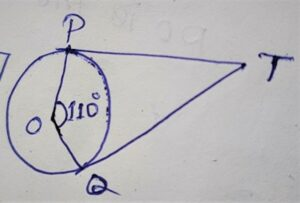∠TPO = ∠TQO = 90º { By theorem 10.1 radius is perpendicular on point of contact to the tangent and circle }

∴ ∠POQ + ∠PTQ = 180º

=> 110º + ∠PTQ  = 180º

=>  ∠PTQ = 180º – 110º

=> ∠PTQ = 70º

Q.3. If tangents P A and P B From a Point P to a circle with center O are inclined to each other at angle of 80º, then ∠POA is equal to

(A) 50º

(B) 60º

(C) 70º

(D) 80º

Explanation:

According to figure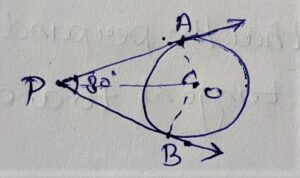∠APB + ∠AOB = 180º

=> 80º + ∠AOB = 180º

=> ∠AOB = 180º – 80º

=> ∠AOB = 100º

=> ∠POA = ∠POB = 100º/2= 50º { ∠AOB/2 }

Q.4. Prove that the tangents drawn at the ends if a diameter of a circle are parallel.

Given: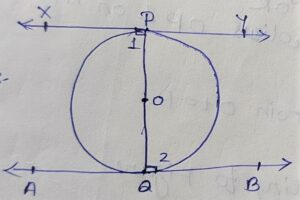According to figure

Circle ( O , r )

X Y and A B are tangents

To Prove:

X Y // A B { X Y parallel to A B }

Construction:

Join points of contacts P and Q to Center O.

Proof:

In figure

∠1 =∠2 { Radii is always perpendicular on point of contacts of tangent By theorem 10.1}

Here ∠1 = ∠2 Which is alternate interior angles and P Q is transversal on X Y and A B

∴ X Y // P Q

Hence the tangents drawn at the ends of a diameter of a circle are parallel.

Q.5. Prove that the perpendicular at the point of contact to the tangent to a circle passes through the center.

Given: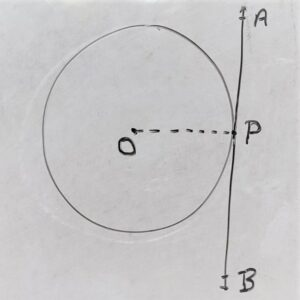According to figure

C (O , r)

A B = tangent which is touches the circle at point P.

∴ P = Point of contact

To Prove:

The Perpendicular at the point of contact to the tangent to a circle passes through the center

Or

O P ⊥ on A B

Construction:

Join point of contact P to center O

Proof:

According to figure

A B = Tangent on circle

P = Point of contact on the circle

We know that by theorem 10.1

“Radius is always ⊥ ( perpendicular) on point of contact of tangent

∴ O P ⊥ on AB

Hence the perpendicular at the point of contact to the tangent to a circle passes through the center.

Q.6. The length of a tangent From a point A at distance 5cm From the center of the circle is 4cm. Find the radius of the circle.

Given: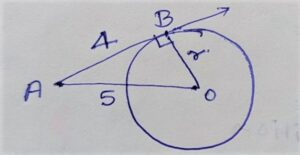According to figure

C ( O, r )

A B = Length of Tangent from point A = 4cm

O A = 5cm

To Find:

The radius of circle OB = r=?

Solution:

In ΔABO

∠ABO = 90º

A B = 4cm

A O = 5cm

O B =?

By PGT

(OA)² = (AB)² + (OB)²

=> (5)² = (4)² + (OB)²

=> 25 = 16 + (OB)²

=> 25 – 16 = (OB)²

=> 9 = (OB)²

=>√9 = OB

=> 3 = OB

Hence Radius of circle = 3cm

Q.7. Two concentric circles are of radii 5cm and 3cm.Find the length of the chord of the larger circle which touches the smaller circle.

Given: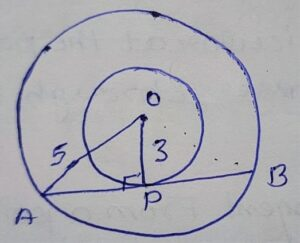According to Figure

Two concentric circles with radius O P = 3cm and O A = 5cm

To Find:

Chord A B =?

Solution:

According to condition

A B = Chord for Larger circle =Tangent for smaller circle

P = Point of contact

Now

In Δ APO

∠APO = 90º

A O = 5 cm

O P = 3cm

A P =?

By PGT

(AO)² = (AP)² + (3)²

=> (5)² = (AP)² + 9

=> 25 – 9 = (AP)²

=> 16 = (AP)²

=> √16 = AP

=> 4 = AP

Radius ( OP ) is always bisect the chord from the point of contact.

A P = P B = 4cm

∴ A B = AP + P B

=> A B = 4 + 4

=> A B = 8cm

Alternate Method:

In Δ APO and ΔBPO

A O = O B { Radii of same circle }

O P = O P {Common side }

∠APO = ∠BPO = 90º

=> Δ APO ≅ Δ BPO { RHS Congruency }

∴ A P = P B { By CPCT }

Hence A P = P B = 4cm

A B = A P + P B

=> A B = 4 + 4

=> A B = 8cm

∴ Length of the chord A B = 8cm.

Q.8. A Quadrilateral ABCD id drawn to circumscribe a circle. (see in figure 10.12)

Prove that A B + C D = A D + B C .

Given: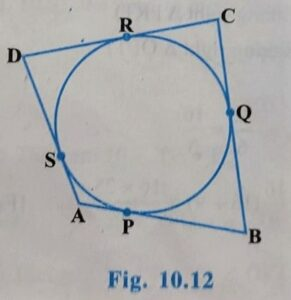According to figure

A B , B C , C D and A D are tangents have point of contacts P , Q , R and S respectively.

To Prove:

A B + C D = A D + B C

Proof:

We know that length of tangent from an external point are equal.

∴  A P = A S ———Equation(i)

B P = B Q ———–Equation (ii)

C R = C Q ———–Equation(iii)

D R = D S ———–Equation(iv)

Adding Equation (i) + Equation (ii)  + Equation(iii) +Equation(iv)

A P + B P + C R + D R = A S + B Q + C Q + D S

=> A B + CD = A S + D S + B Q + C Q

=> A B + C D = A D + B C

Hence Proved

Q.9. In Figure 10.13, X Y and X’ Y’ are two parallel tangents to a circle with center  O and another tangent A B with point of contact  C intersecting X Y at A and X’ Y’ at B. Prove that ∠AOB = 90º

Given: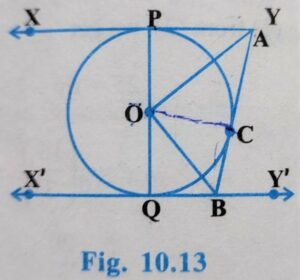According to figure

diagram

tangents X Y // X’ Y’

To Prove:

∠AOB = 90º

Construction:

Join O to C

Proof:

By Theorem 10.2 :We know that “Length of tangent Drawn From an External point are equal in Length”.

P A = A C ———– Equation (i)

In ΔQBO and ΔBCO

∠BQO = ∠BCO = 90º { By Theorem 10.1 Radius is always ⊥ on point of contact }

O B = O B {common side }

O Q =  O C { Radii of same circle }

ΔQBO ≅ Δ BCO { By RHS Congruence }

∠1 = ∠2 ——————–(i)

Similarly

In Δ PAO and Δ CAO

P A = C A { From Equation (i) }

A O = A O { common side }

O P = O C { Radii of same circle }

∴ Δ PAO ≅ Δ CAO { By SSS congruence }

∠ PAO = ∠ CAO { By CPCPT }

=> ∠3 = ∠5 ———————(ii)

We know that sum of angles subtended same side on a line at a point are 180º

∠1 + ∠2 + ∠3 + ∠5 = 180°

=> ∠2 + ∠2 + ∠3 + ∠3 = 180º

=> 2∠2 + 2∠3 = 180º

=> 2(∠2 + ∠3) = 180º

=> ∠2 + ∠3 = 180º/2

=> ∠2 + ∠3 = 90º

=> ∠AOB = 90º

Hence Proved

Q.10. Prove that the angle between the two tangents drawn from an external point to a circle is supplementary to the angle subtended by the line – segment joining the points  of contact at the center .

Given:

According to figure

di

P A and P B are tangents from an external point P

To Prove:

∠APB + ∠AOB = 180

Proof:

In figure

∠PAO = ∠PBO = 90º { Radii is always ⊥ on point of contact }

∠APB + ∠PAO + ∠AOB + ∠PBO = 360º

=> ∠APB + 90º + ∠AOB + 90° = 360º

=> ∠APB + ∠AOB + 180º = 360º

=> ∠APB + ∠AOB = 360º – 180º

=> ∠APB + ∠AOB = 180º

Hence Proved

Q.11. Prove that the parallelogram circumscribing a circle is rhombus.

Given:

According to figure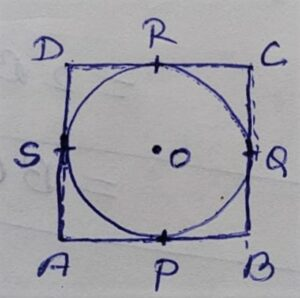A B // D C and A B =C D

A D // B C and A D = B C

Quadrilateral ABCD is circumscribing a circle with center O

To Prove:

Parallelogram ABCD is a rhombus

OR

A B = B C = C D = A D

Proof:

According to figure:

We know that By theorem 10.2 “Length of tangents drawn from an external point are equal”

B P = B Q ———–Equation(i)

A P = A S ————Equation (ii)

C R = C Q ———–Equation (iii)

D R = D S ———–Equation (iv)

Adding Equation (i) + Equation (ii) + Equation (iii) + Equation (iv)

B P + A P + C R + D R = B Q + A S + C Q + D S

=> A B + C D =  B Q + C Q + A S + D S

=> A B + C D = B C + A D

=> 2 A B = 2 B C

=> A B = B C

∴ A B = B C = C D = A D

Hence parallelogram ABCD is a rhombus.

Q.12. A Triangle ABC is drawn to circumscribe a circle of radius 4cm such that the segments B D and D C into which B C is divided by the point of contact D are of length 8cm and 6cm respectively (see fig. 10.14).Find the sides A B and A C.

Given: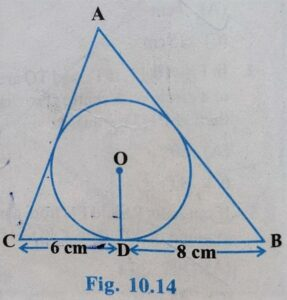In ΔABC:

ΔABC is drawn to circumscribe a circle if radius 4cm

To Find:

Length of side A B = ?

Length of side A C =?

Construction:

Join vertices A , B and C to center OBC = 6 + 8 = 14 cm

We know that tangents drawn from an external point are equal in length.

∴  A F = A E = X (let)

C D = C F = 6cm

B D = B E = 8cm

a = A B = (x + 8 );

b = B C = 14 cm

c = A C = (6+x)

By Heron’s Formula

S = (A B + B C + A C)/2

=> S =(a + b + c)/2

=> S = ( x + 8 + 14 + 6 + x )/2

=> S = ( 2x +  28 )/2

=> S = 2(x + 14)/2

=> S = 2(x + 14)/2

=> S = x + 14

s – a = x + 14 -( x + 8)

=> s – a = x + 14 – x – 8

=> s – a = 6

s – b = x + 14 – 14

=> s – b = x

s – c = x + 14 – ( x + 6)

=> s – c = x + 14 – x – 6

=> s – c = 8

Area of Δ ABC = √s(s-a)(s-b)(s-c)

=> Area of Δ ABC = √(x+14)(x)(6)(8)

=> Area of  Δ ABC = √(x+14)48x  ————–Equation(i)

Area of Δ ABC  = Area of Δ AOB + Area of Δ BOC + Area of Δ AOC

=> Area of Δ ABC  = 1/2 × b × h + 1/2 × b × h + 1/2 × b × h

=> Area of Δ ABC = 1/2 × A B × 4 + 1/2 × B C × 4 + 1/2 × A C × 4

=> Area of Δ ABC = 1/2 ×4 ( A B + B C + A C )

=> Area of Δ ABC = 2 ( A B + B C + A C )

=> Area of Δ ABC = 2 ( 8 + x + 14 + x + 6 )

=> Area of Δ ABC = 2 (2x + 28)

=> Area of Δ ABC = 2×2(x + 14)

=> Area of Δ ABC = 4 ( x + 14) ———-Equation(ii)

Equation (i) = Equation (ii)

Area of  Δ ABC = Area of  Δ ABC

√(x+14)48x = 4 ( x + 14)

squaring both side

=> (x+14)48x = 16 ( x + 14)²

=> 48x = 16 ( x + 14)²/(x+14)

=> 48x = 16(x + 14)

=> 48x/16 = (x+ 14)

=> 3x = x + 14

=> 3x – x = 14

=> 2x = 14

=> x = 14/2

=> x = 7

Hence

A B = x + 8 = 7 + 8 = 15cm

B C = x + 6 = 6 + 7 = 13cm

Q.13. Prove that opposite sides of a quadrilateral circumscribing a circle subtend supplementary angle at the center of the circle.

Given:

According to Diagram: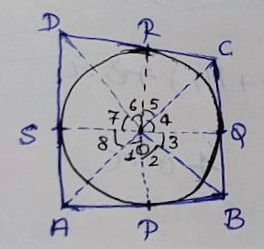ABCD is a quadrilateral circumscribing a circle with center O

tangents A B , B C , C D , and A  D have point of contact P, Q, R and S respectively.

To Prove:

∠AOB + ∠COD = 180º

∠AOD + ∠BOC = 180º

Proof:

Angle subtend by the tangents external point on the center of circle are equal.

∠2 = ∠3 ——-Equation(i)

∠1 = ∠8 ——-Equation(ii)

∠4 = ∠5 ——-Equation(iii)

∠6 = ∠7 ——-Equation(iv)

We know that sum of all angles subtended on a point are 360°

∴ ∠1 + ∠2 + ∠3 + ∠4 + ∠5 + ∠6 + ∠7 + ∠8 = 360º

=> ∠1 + ∠2 + ∠2 + ∠5 + ∠5 + ∠6 + ∠6 + ∠1 = 360º { From Equation (i),(ii),(iii) and (iv) }

=> 2∠1 + 2∠2 + 2∠5 + 2∠6  = 360°

=> 2(∠1 + ∠2 + ∠5 + ∠6) = 360º

=> 2 ( ∠AOB + ∠COD ) = 360° { ∠1 + ∠2 = ∠AOB ; ∠5 + ∠6 = ∠COD }

=> ∠AOB + ∠COD = 360°/2

=> ∠AOB + ∠COD = 180º

Similarly

∠AOD + ∠BOC = 180º

Hence Opposite sides of a quadrilateral circumscribing a circle subtend supplementary angles at the center of the circle.